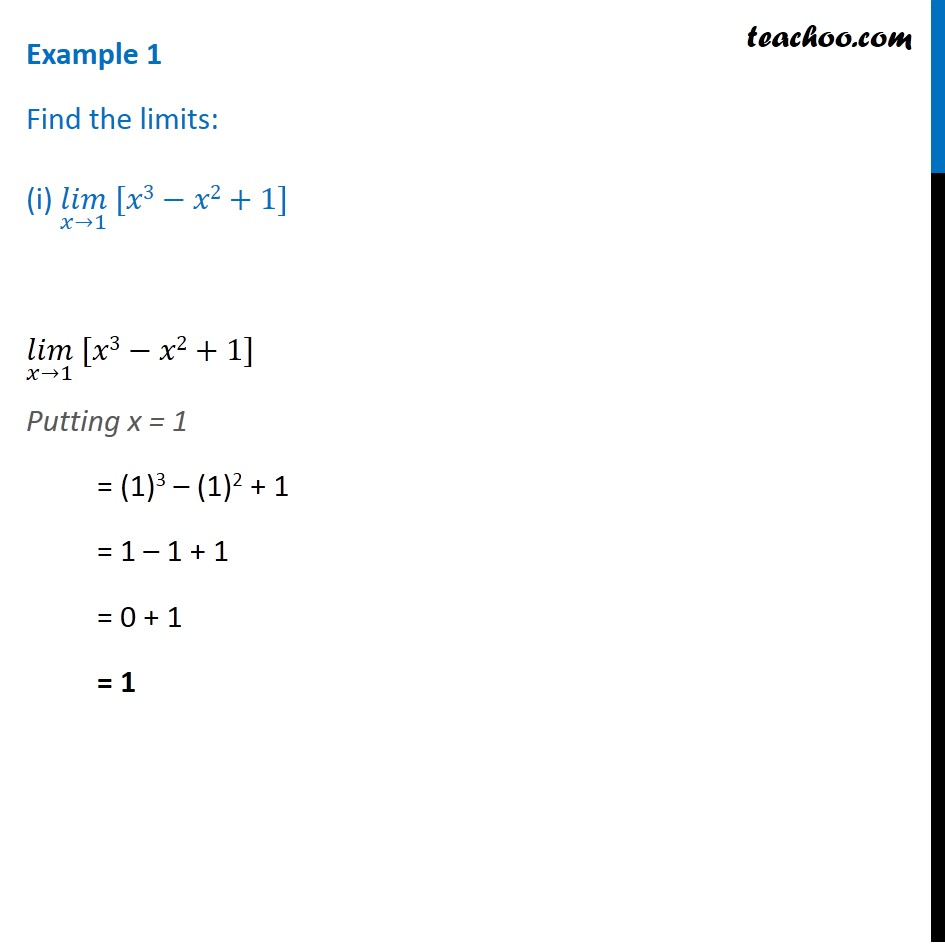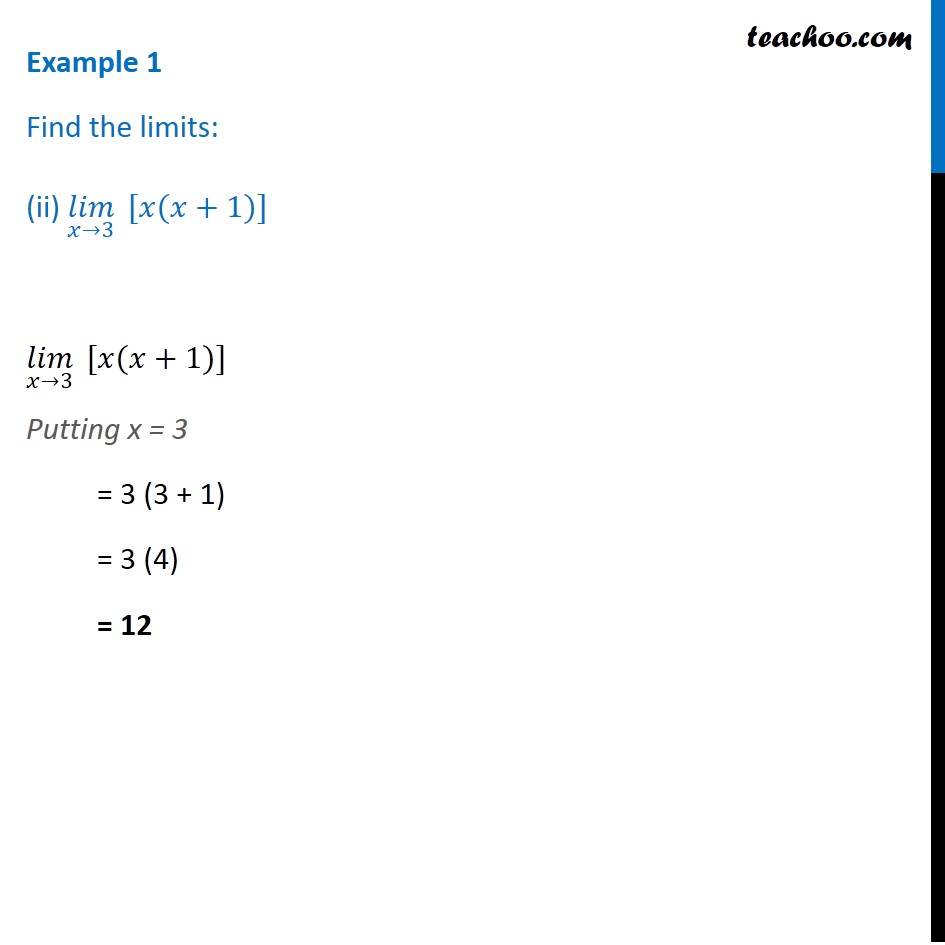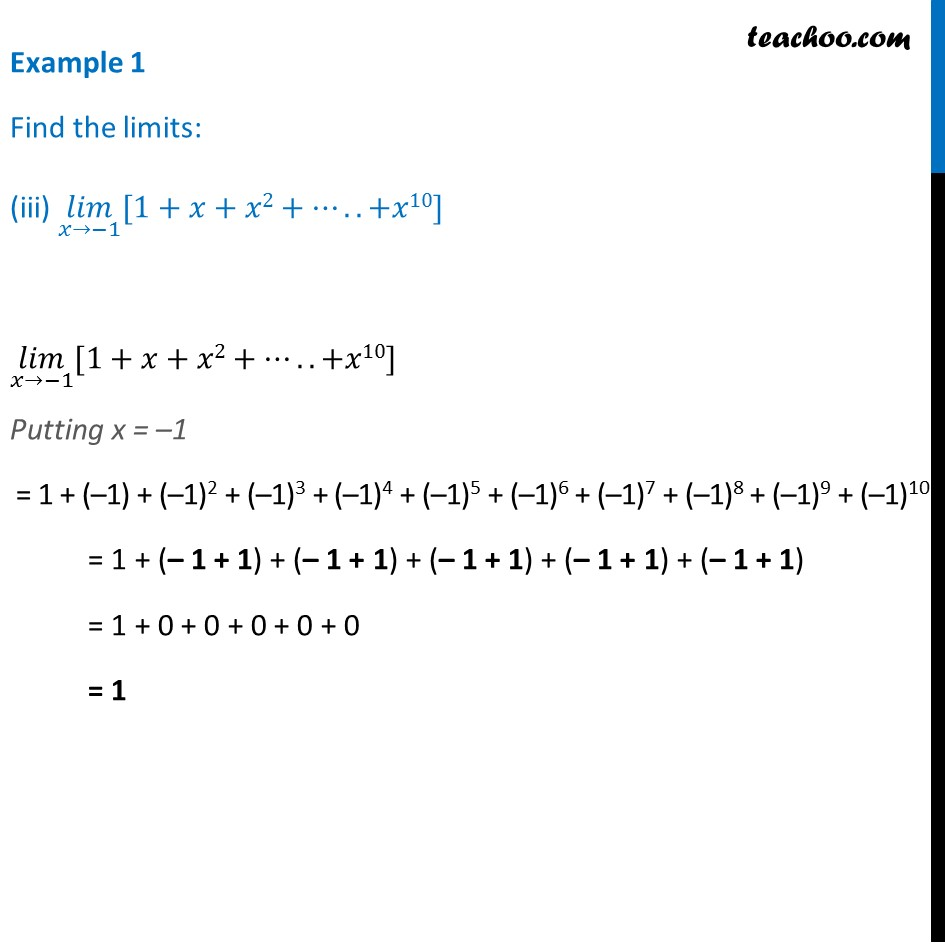1. Chapter 13 Class 11 Limits and Derivatives
2. Concept wise
3. Limits - Definition

Transcript

Example 1 Find the limits: (i) 〖(𝑙𝑖𝑚)┬(𝑥→1) 〗⁡〖[𝑥3−𝑥2+1]〗 〖(𝑙𝑖𝑚)┬(𝑥→1) 〗⁡〖[𝑥3−𝑥2+1]〗 Putting x = 1 = (1)3 – (1)2 + 1 = 1 – 1 + 1 = 0 + 1 = 1 Example 1 Find the limits: (ii) (𝑙𝑖𝑚)┬(𝑥→3)⁡〖 [𝑥(𝑥+1)]〗 (𝑙𝑖𝑚)┬(𝑥→3)⁡〖 [𝑥(𝑥+1)]〗 Putting x = 3 = 3 (3 + 1) = 3 (4) = 12 Example 1 Find the limits: (iii) (𝑙𝑖𝑚)┬(𝑥→−1)⁡〖[1+𝑥+𝑥2+…..+𝑥10]〗 (𝑙𝑖𝑚)┬(𝑥→−1)⁡〖[1+𝑥+𝑥2+…..+𝑥10]〗 Putting x = –1 = 1 + (–1) + (–1)2 +…….+ (–1)10 = 1 – 1 + 1 + …….. + 1 = 0 + 0 + 0 + ……..0 = 1

Limits - Definition